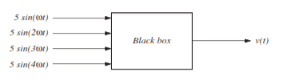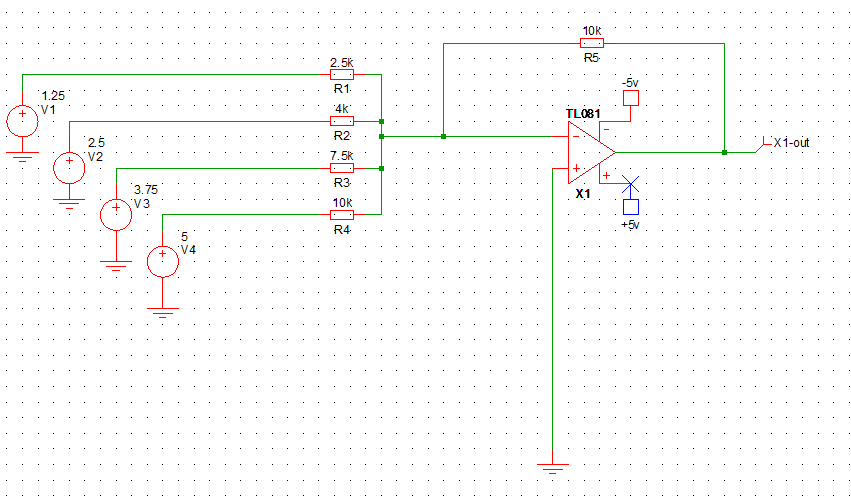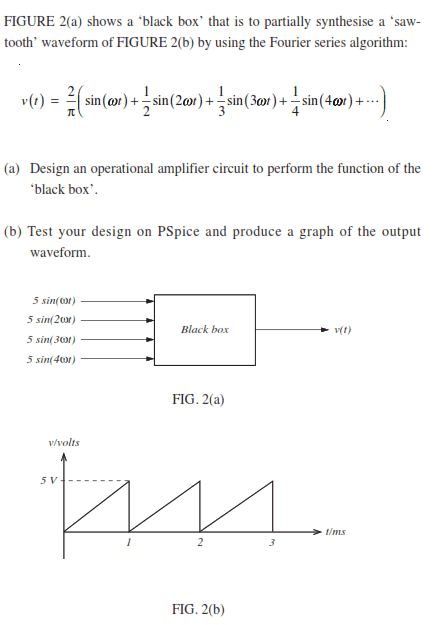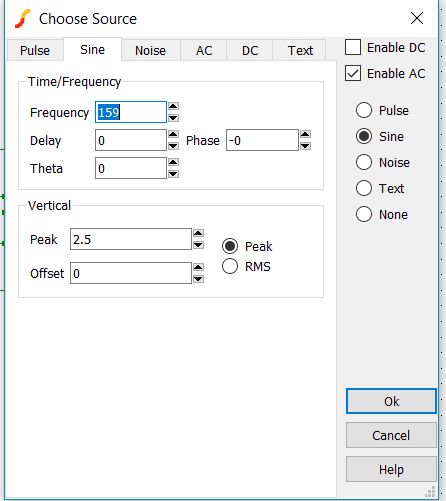# Black-box design to create sawtooth waveform

• Engineering
peasngravy
Homework Statement:
FIGURE 2(a) shows a ‘black box’ that is to partially synthesise a ‘sawtooth’ waveform of FIGURE 2(b) by using the Fourier series
algorithm:
Relevant Equations:
v(t) = 2/pi(sin.omega.t)
Hi

I received some great help yesterday and i was hoping someone would be able to help me out again today

Note: the given equation is very long and would look very messy without being typed in the correct format so I didn't enter the entire thing in the relevant equations box; instead it is included in the screenshot belowI am not sure at all what is being asked of me here. There are no Fourier algorithms in any of my notes so I am struggling with what I can do with this.

What I know so far is that the amplitude is 5 volts, and the frequency is 1000hz (1 cycle per ms on the graph).

Could someone please explain how I should use the Fourier algorithm to find my answer?

Thanks

Mentor
Homework Statement:: FIGURE 2(a) shows a ‘black box’ that is to partially synthesise a ‘sawtooth’ waveform of FIGURE 2(b) by using the Fourier series
algorithm:
Relevant Equations:: v(t) = 2/pi(sin.omega.t)

Hi

I received some great help yesterday and i was hoping someone would be able to help me out again today

Note: the given equation is very long and would look very messy without being typed in the correct format so I didn't enter the entire thing in the relevant equations box; instead it is included in the screenshot below

View attachment 271794

I am not sure at all what is being asked of me here. There are no Fourier algorithms in any of my notes so I am struggling with what I can do with this.

What I know so far is that the amplitude is 5 volts, and the frequency is 1000hz (1 cycle per ms on the graph).

Could someone please explain how I should use the Fourier algorithm to find my answer?

Thanks
Yoiks! My first inclination would be to use an "integrate and dump" circuit; I'm not sure what they want you to do with the FS approximation and opamps. Are you allowed to use transistors too, or just opamps and passive components?

•peasngravy
peasngravy
It doesn't really state either way but I would suspect just passives and opamps. It really does seem a few levels above the rest of the questions

Mentor
Step back a bit and take a broader look at it.The sine functions are already given as inputs to the box. The "formula" is a weighted sum of them...

•peasngravy and berkeman
peasngravy
So the formulas then become
5sin(1000*t)
5sin(2*1000*t) and so on?

t is obviously time, but what time do I need to use for these figures?

Mentor
Step back a bit and take a broader look at it.
View attachment 271811
The sine functions are already given as inputs to the box. The "formula" is a weighted sum of them...
Oh jeeze, I missed that. Thanks!

Mentor
So the formulas then become
5sin(1000*t)
5sin(2*1000*t) and so on?

t is obviously time, but what time do I need to use for these figures?
As gneill says, you are provided the sinusoidal signals as inputs. It looks like you just need to scale each input appropriately and combine them... Can you say what kinds of opamp circuits would be used for each of those functions? And are there ways to simplify it down to just a few or one opamp?

•peasngravy and gneill
peasngravy
As gneill says, you are provided the sinusoidal signals as inputs. It looks like you just need to scale each input appropriately and combine them... Can you say what kinds of opamp circuits would be used for each of those functions? And are there ways to simplify it down to just a few or one opamp?

I think it will just be a very basic summing amplifier

I did find this page which looks like it may be of some help for the circuit http://www.ecircuitcenter.com/Circuits/opsum/opsum2.htm

But I don't even know where to begin with those sinusoidal signals as an input - could you give me a couple of pointers on what I should do there?

It's the time part I am unsure about. How do I know what value of time to put into the equation? Do I just pick the time it takes for one cycle, i.e. 0.001 seconds?

Last edited:
Mentor
I think it will just be a very basic summing amplifier
That sounds right, but I'd like to see your attempt at including the scaling in that summing amplifier...
I did find this page which looks like it may be of some help for the circuit http://www.ecircuitcenter.com/Circuits/opsum/opsum2.htm
That's close, but it's an inverting topology summing amplifier. You can look for a non-inverting version or follow that one with a unity-gain inverting opamp stage...
It's the time part I am unsure about. How do I know what value of time to put into the equation? Do I just pick the time it takes for one cycle, i.e. 0.001 seconds?
What I know so far is that the amplitude is 5 volts, and the frequency is 1000hz
To draw the opamp circuit, you don't need to worry about that. I'm guessing you are asking about the simulation part of the question. To be honest, I don't know offhand if you want ##\frac{\omega}{2\pi}## to be the 1kHz frequency or if you want twice or half of that. It's easy to just try the different values in your simulation to verify which choice gives you the right waveform.

(You could also look up the Fourier Series expansion of that waveform to see what value of ##\omega## then are using. I'm too lazy to do that right now...)•peasngravy
peasngravy
I was asking about those as I thought I may need that to work out the input voltages at each resistor at the input. I suppose I could go for 1v and see what happens

I am going to give this a go tomorrow in that case, as it's getting late here. I will start off with a 4 resistors, 1k, 2k, 3k and 4k and use a bit of trial and error from there. I will keep reporting back as no doubt I will get stuck again... :)

•berkeman
Gold Member
t is obviously time, but what time do I need to use for these figures?

Since analog devices are continuous-time devices (within their bandwidth), you don't need to worry about the "t" in the Fourier representation, it disappears; i.e. it becomes an infinite sum of infinitesimals, which ends up being unity here.

peasngravy
I am having a go at this now without too much luck.

I tried calculating 4voltage inputs, with the equations 5sin(1000), 5sin(1000*2), 5sin(1000*3) and 5sin(1000*4) as I didn't really know what else to do. I made each of these signals 1000, 2000, 3000 and 4000hz

Mentor
Don't worry about specific input voltage values for a particular instant in time. The black box circuit won't contain any time-dependent components like capacitors or inductors. It's simply a summing circuit. You just want to have the summing inputs have the appropriate weights (gains).

The time-dependent part comes when you simulate the system with Spice. It provides built-in voltage sources where you can simply supply the required voltage and frequency, and everything is then handled for you.

peasngravy
So where I've got to is this:

As there are 4 inputs then ωt, time interval, is 0.25ms

So
5sin(ωt) = 5sin^-1 (0.25) = 1.25v, 14.5°
5sin(2ωt) = 5sin^-1 (2*0.25) = 2.5v, 30°
5sin(3ωt) = 5sin^-1 (3*0.25) = 3.75v, 48.6°
5sin(4ωt) = 5sin^-1 (4*0.25) = 5v, 90°

Gain = Vout/Vin = Rf/Rin

Gain of 1st input = 5/1.25 = 4 = rf/rin
If i make Rf 10k, then Rin = 10k/4 = 2.5k

And follow this on for the rest of the inputs - am i on the right track?

PS - is there a way to type equations so they appear correctly as fractions?

Mentor
PS - is there a way to type equations so they appear correctly as fractions?
Yes. Click on the "LaTeX Guide" link below the Edit window to see how to type equations here.

You can also click Reply on my post to see how I wrote this fraction:

Gain of 1st input = ## \frac{5}{1.25} = 4 = \frac{r_f}{r_{in}}##

(or on a separate line): $$\frac{5}{1.25} = 4 = \frac{r_f}{r_{in}}$$

Mentor
So
5sin(ωt) = 5sin^-1 (0.25) = 1.25v, 14.5°
5sin(2ωt) = 5sin^-1 (2*0.25) = 2.5v, 30°
5sin(3ωt) = 5sin^-1 (3*0.25) = 3.75v, 48.6°
5sin(4ωt) = 5sin^-1 (4*0.25) = 5v, 90°
I don't think you need that for anything. Can you show us the opamp schematic with the resistors labeled? Have you started drawing your simulation circuit yet?

•peasngravy
Mentor
this is my schematic so far with those gains, but it doesn't have the correct waveform yet

View attachment 271849
The input voltages should all have an amplitude of 5V, since those are the inputs to your block. And this is still an inverting topology, so to get the correct waveform you will need to invert the output (and maybe add an offset?)... Oh, and you will need wider power supply rails than just +/-5V, especially with a TL081 opamp -- Can you say why?peasngravy
Oops I just checked the TL 081 requirements and it needs a supply of +/-7-36V

Mentor
Oops I just checked the TL 081 requirements and it needs a supply of +/-7-36V
Yeah, it's definitely not a rail-to-rail opamp, but it should be fine for this simulation. I'd just set its rails to +/-15V to give plenty of headroom.

peasngravy
I changed this now - I am just learning about op-amps now so I will make many more of these silly mistakes, unfortunately!

I changed that, and flipped the op-amp around, but I get nothing like what I am looking for. I added a dc offset at the inverting terminal.

I feel like I am way out of my depth with this right now :(

Mentor
I changed that, and flipped the op-amp around, but I get nothing like what I am looking for. I added a dc offset at the inverting terminal.
Can you post your updated schematic? And be careful -- the gain of a non-inverting summing stage is different from that of an inverting stage...

peasngravy
Offset is set to -6 right now but I've just been messing around with different values for it for now

just editing to add - op amp rail supply is now +- 15, i just forgot to change the name of the terminals

Last edited:
peasngravy
I just want to say i appreciate all the help you have given me so far. The theory side of this stuff is all new to me

•berkeman
Mentor
You're welcome. We all learned this stuff at one point.Hopefully you have some good references for opamps, but here is one that looks to be a good intro and summary:

https://www.nutsvolts.com/magazine/article/op-amp-cookbook

peasngravy
So if i want the sum of the 4 inputs to be 5v, with a 5 volt input to each one, I would need them all to have a gain of -4 right, giving 4 inputs of 1.25V making a total Vin of 5v?

So a feedback resistor of 10k, with each input resistor being 2.5k, this would give me a gain of -4?

As Gain = Vin/Vout = -(Rf/Rin)

Mentor
So if i want the sum of the 4 inputs to be 5v, with a 5 volt input to each one, I would need them all to have a gain of -4 right, giving 4 inputs of 1.25V making a total Vin of 5v?

So a feedback resistor of 10k, with each input resistor being 2.5k, this would give me a gain of -4?

As Gain = Vin/Vout = -(Rf/Rin)
The gains for each component (sin waves of different frequencies) are given in your first post as the Fourier Series coefficients...peasngravy
I see - so those 4 voltages I calculated from the sin functions earlier should be how I calculate the gains i need?

i.e. 5/1.25
5/2.5
5/3.75
and 5/5 ?

Mentor
I see - so those 4 voltages I calculated from the sin functions earlier should be how I calculate the gains i need?

i.e. 5/1.25
5/2.5
5/3.75
and 5/5 ?
Something like that. Once you get the sawtooth waveform, you can fine tune the coefficients to get the 2.5V peak amplitude (plus remember to include the 2.5V constant offset term, both in the FW (which they omitted in the problem statement) and in your opamp circuit).

#### Attachments

peasngravy
These need to be AC supplies with set frequencies right? otherwise i'll never get a waveform

Mentor
Yes, the diagram shows a peak amplitude of 5V and you said the bade frequency is 1kHz. You increase the frequencies of each AC Source as shown in the FS. Can you paste a screenshot of your AC Source definition for the first one?

•peasngravy
peasngravy
Yes, the diagram shows a peak amplitude of 5V and you said the bade frequency is 1kHz. You increase the frequencies of each AC Source as shown in the FS. Can you paste a screenshot of your AC Source definition for the first one?(i spent far longer than I should have trying to post this reply by hitting OK on the screenshot)

Mentor
From the problem statement figure, you want the frequency of the first one to be ##2\pi f## where f=1kHz, the frequency of the 2nd source to be twice that, and so on. And the peak amplitude of each is 5V, no?

Mentor
BTW, that Fourier Series for the sawtooth wave doesn't look quite right to me, but I'm looking into that more now. If you can post your circuit after setting up the 4 AC Sources and the output waveform that it generates, that would be a help.